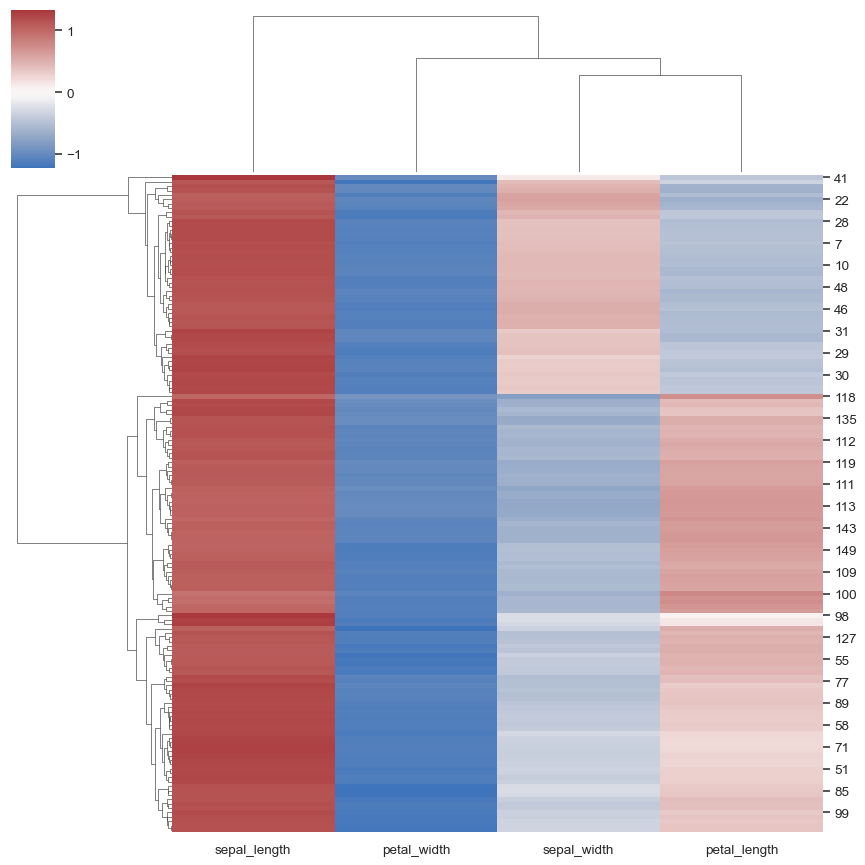# seaborn.clustermap#

seaborn.clustermap(data, *, pivot_kws=None, method='average', metric='euclidean', z_score=None, standard_scale=None, figsize=(10, 10), cbar_kws=None, row_cluster=True, col_cluster=True, row_linkage=None, col_linkage=None, row_colors=None, col_colors=None, mask=None, dendrogram_ratio=0.2, colors_ratio=0.03, cbar_pos=(0.02, 0.8, 0.05, 0.18), tree_kws=None, **kwargs)#

Plot a matrix dataset as a hierarchically-clustered heatmap.

This function requires scipy to be available.

Parameters:
data2D array-like

Rectangular data for clustering. Cannot contain NAs.

pivot_kwsdict, optional

If `data` is a tidy dataframe, can provide keyword arguments for pivot to create a rectangular dataframe.

methodstr, optional

Linkage method to use for calculating clusters. See `scipy.cluster.hierarchy.linkage()` documentation for more information.

metricstr, optional

Distance metric to use for the data. See `scipy.spatial.distance.pdist()` documentation for more options. To use different metrics (or methods) for rows and columns, you may construct each linkage matrix yourself and provide them as `{row,col}_linkage`.

z_scoreint or None, optional

Either 0 (rows) or 1 (columns). Whether or not to calculate z-scores for the rows or the columns. Z scores are: z = (x - mean)/std, so values in each row (column) will get the mean of the row (column) subtracted, then divided by the standard deviation of the row (column). This ensures that each row (column) has mean of 0 and variance of 1.

standard_scaleint or None, optional

Either 0 (rows) or 1 (columns). Whether or not to standardize that dimension, meaning for each row or column, subtract the minimum and divide each by its maximum.

figsizetuple of (width, height), optional

Overall size of the figure.

cbar_kwsdict, optional

Keyword arguments to pass to `cbar_kws` in `heatmap()`, e.g. to add a label to the colorbar.

{row,col}_clusterbool, optional

If `True`, cluster the {rows, columns}.

{row,col}_linkage`numpy.ndarray`, optional

Precomputed linkage matrix for the rows or columns. See `scipy.cluster.hierarchy.linkage()` for specific formats.

{row,col}_colorslist-like or pandas DataFrame/Series, optional

List of colors to label for either the rows or columns. Useful to evaluate whether samples within a group are clustered together. Can use nested lists or DataFrame for multiple color levels of labeling. If given as a `pandas.DataFrame` or `pandas.Series`, labels for the colors are extracted from the DataFrames column names or from the name of the Series. DataFrame/Series colors are also matched to the data by their index, ensuring colors are drawn in the correct order.

If passed, data will not be shown in cells where `mask` is True. Cells with missing values are automatically masked. Only used for visualizing, not for calculating.

{dendrogram,colors}_ratiofloat, or pair of floats, optional

Proportion of the figure size devoted to the two marginal elements. If a pair is given, they correspond to (row, col) ratios.

cbar_postuple of (left, bottom, width, height), optional

Position of the colorbar axes in the figure. Setting to `None` will disable the colorbar.

tree_kwsdict, optional

Parameters for the `matplotlib.collections.LineCollection` that is used to plot the lines of the dendrogram tree.

kwargsother keyword arguments

All other keyword arguments are passed to `heatmap()`.

Returns:
`ClusterGrid`

A `ClusterGrid` instance.

`heatmap`

Plot rectangular data as a color-encoded matrix.

Notes

The returned object has a `savefig` method that should be used if you want to save the figure object without clipping the dendrograms.

To access the reordered row indices, use: `clustergrid.dendrogram_row.reordered_ind`

Column indices, use: `clustergrid.dendrogram_col.reordered_ind`

Examples

Plot a heatmap with row and column clustering:

```iris = sns.load_dataset("iris")
species = iris.pop("species")
sns.clustermap(iris)
```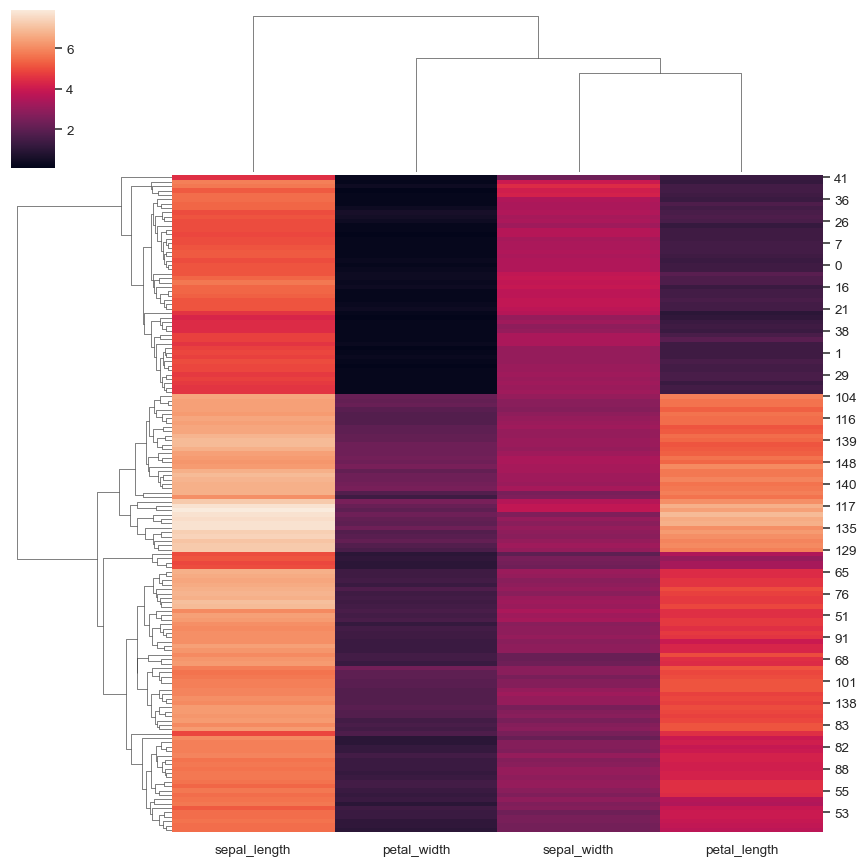Change the size and layout of the figure:

```sns.clustermap(
iris,
figsize=(7, 5),
row_cluster=False,
dendrogram_ratio=(.1, .2),
cbar_pos=(0, .2, .03, .4)
)
```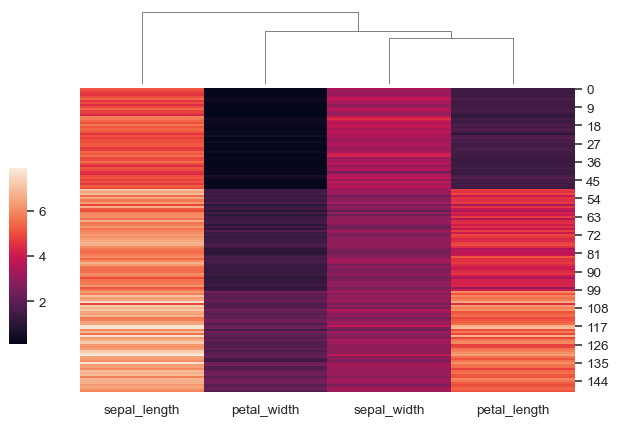Add colored labels to identify observations:

```lut = dict(zip(species.unique(), "rbg"))
row_colors = species.map(lut)
sns.clustermap(iris, row_colors=row_colors)
```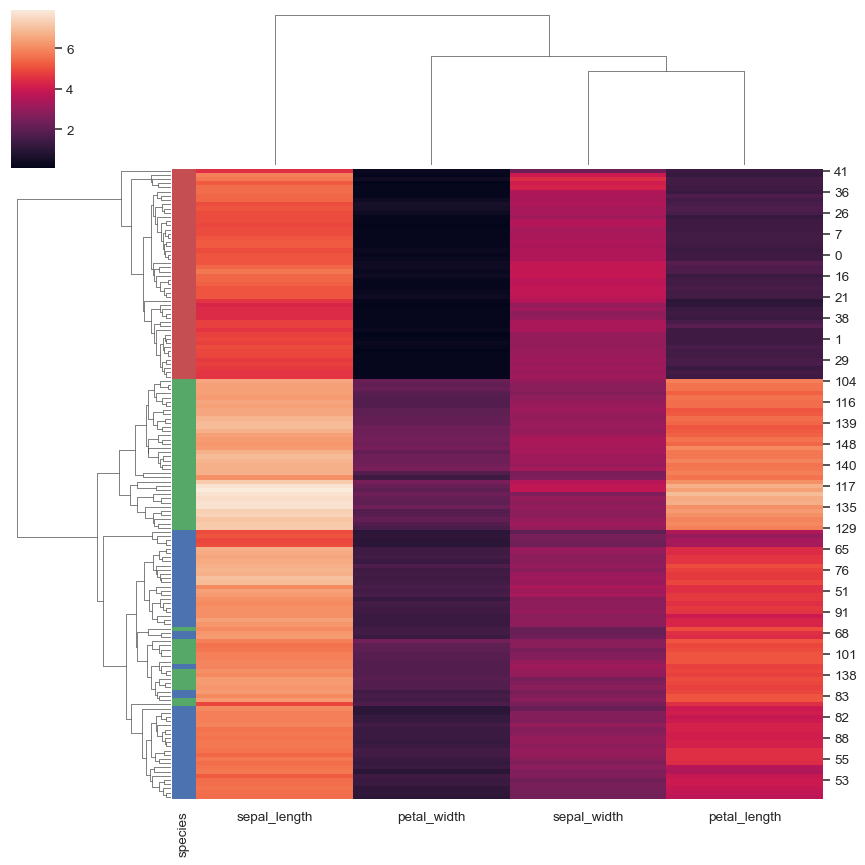Use a different colormap and adjust the limits of the color range:

```sns.clustermap(iris, cmap="mako", vmin=0, vmax=10)
```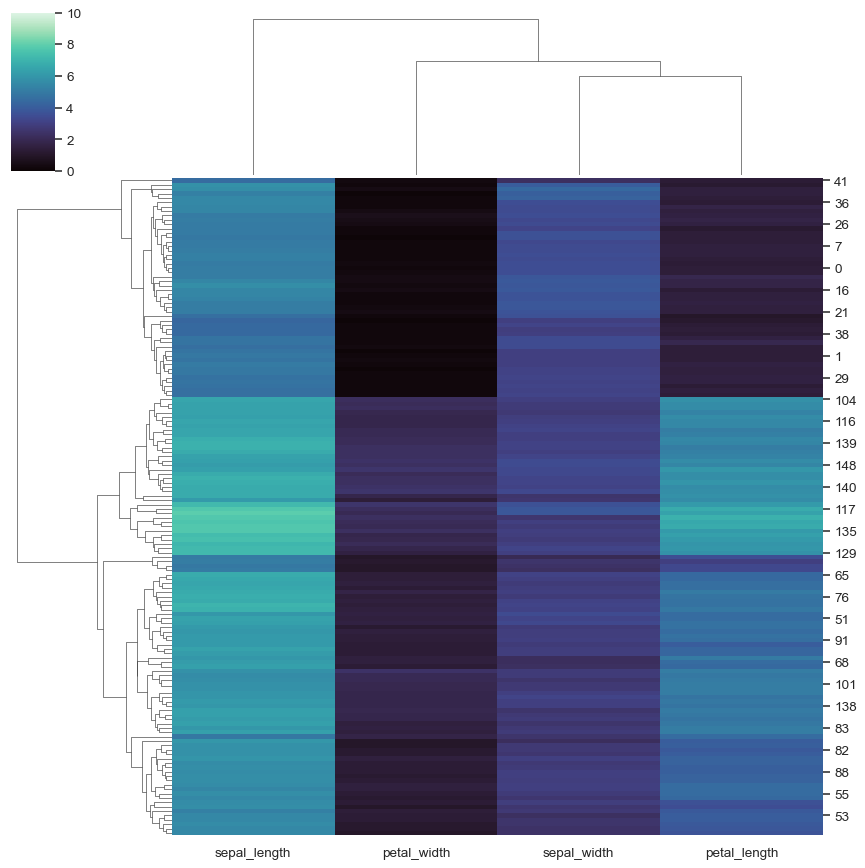Use differente clustering parameters:

```sns.clustermap(iris, metric="correlation", method="single")
```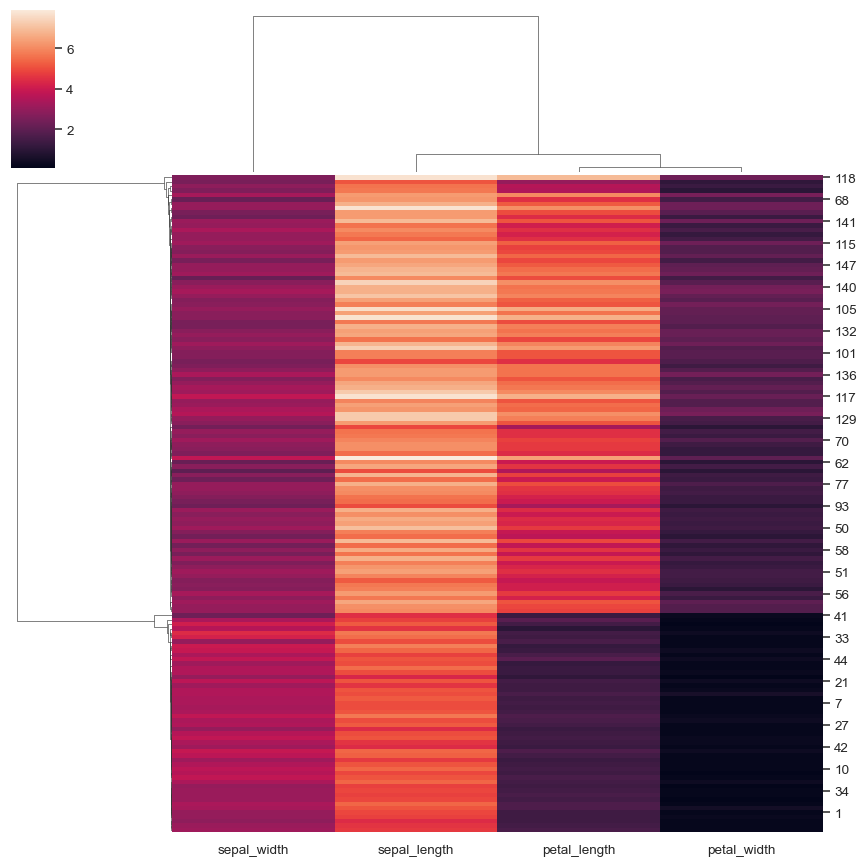Standardize the data within the columns:

```sns.clustermap(iris, standard_scale=1)
```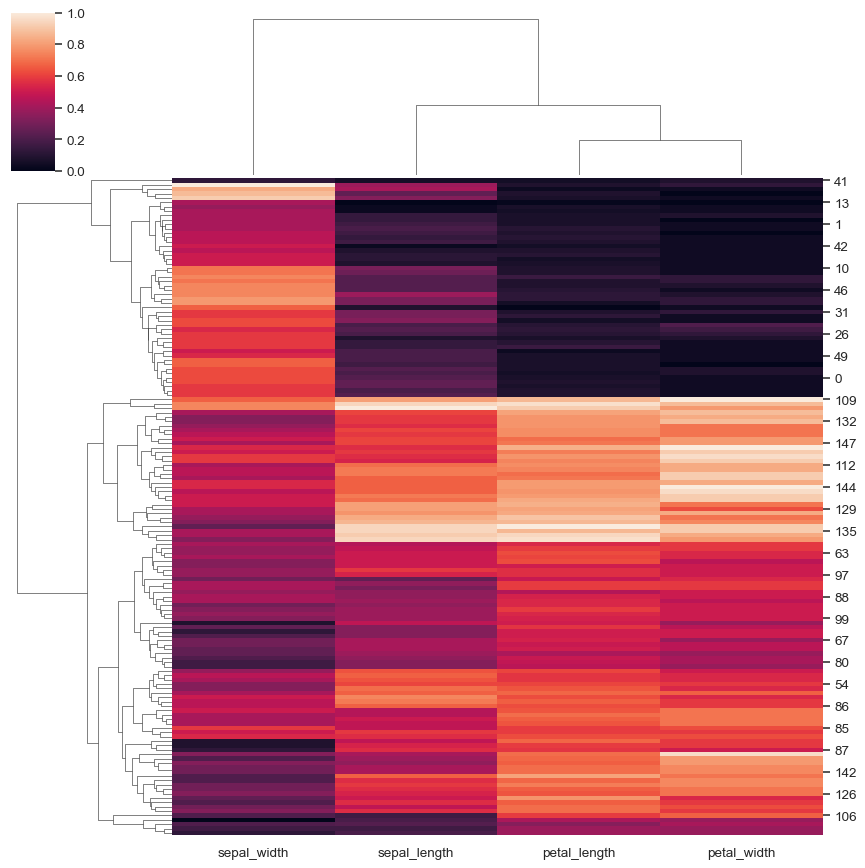Normalize the data within rows:

```sns.clustermap(iris, z_score=0, cmap="vlag", center=0)
```